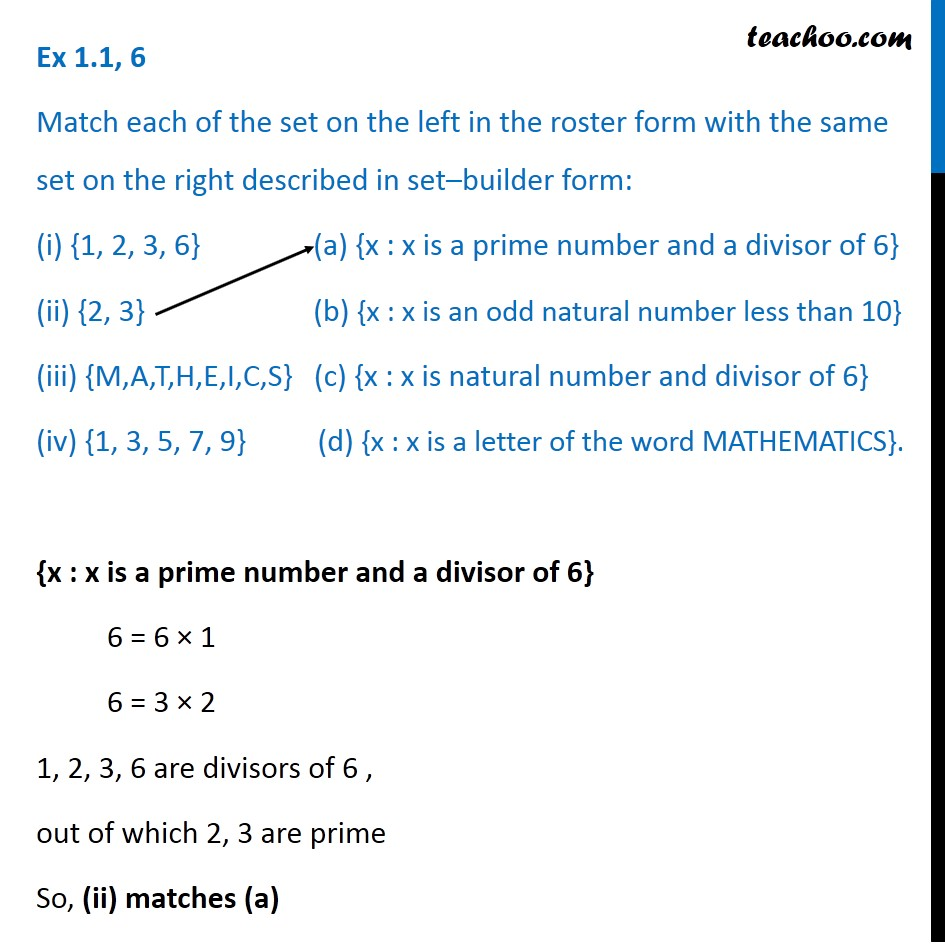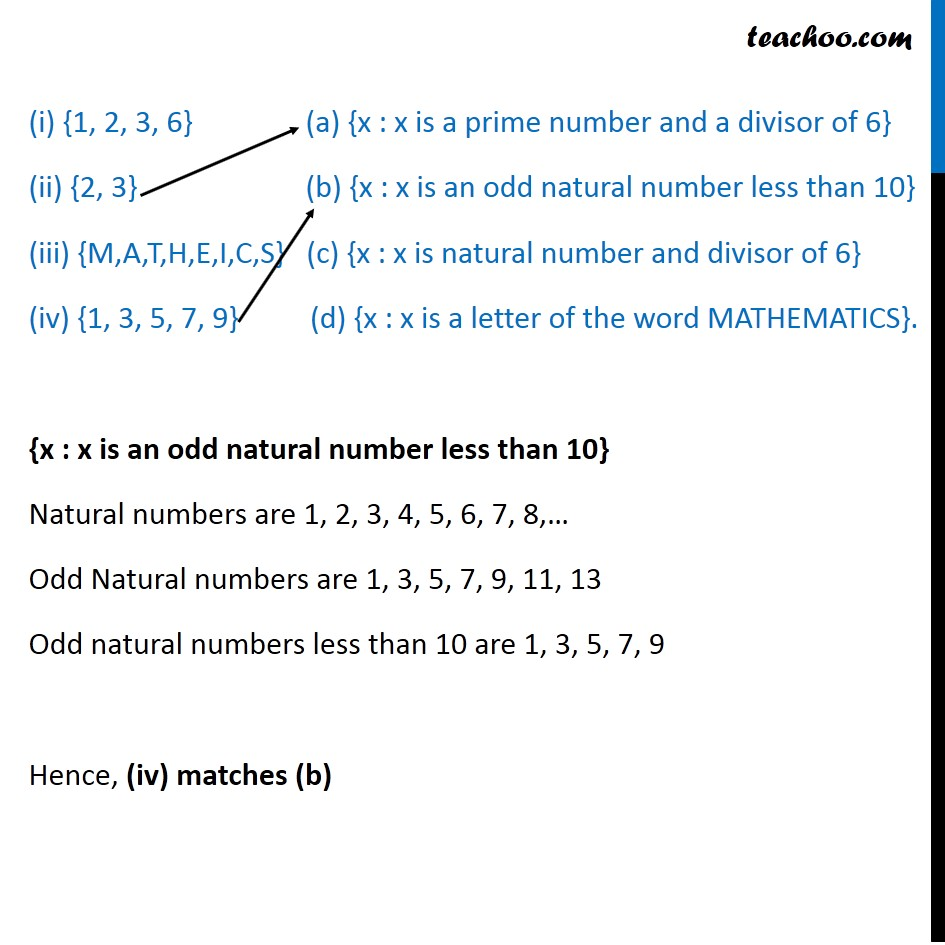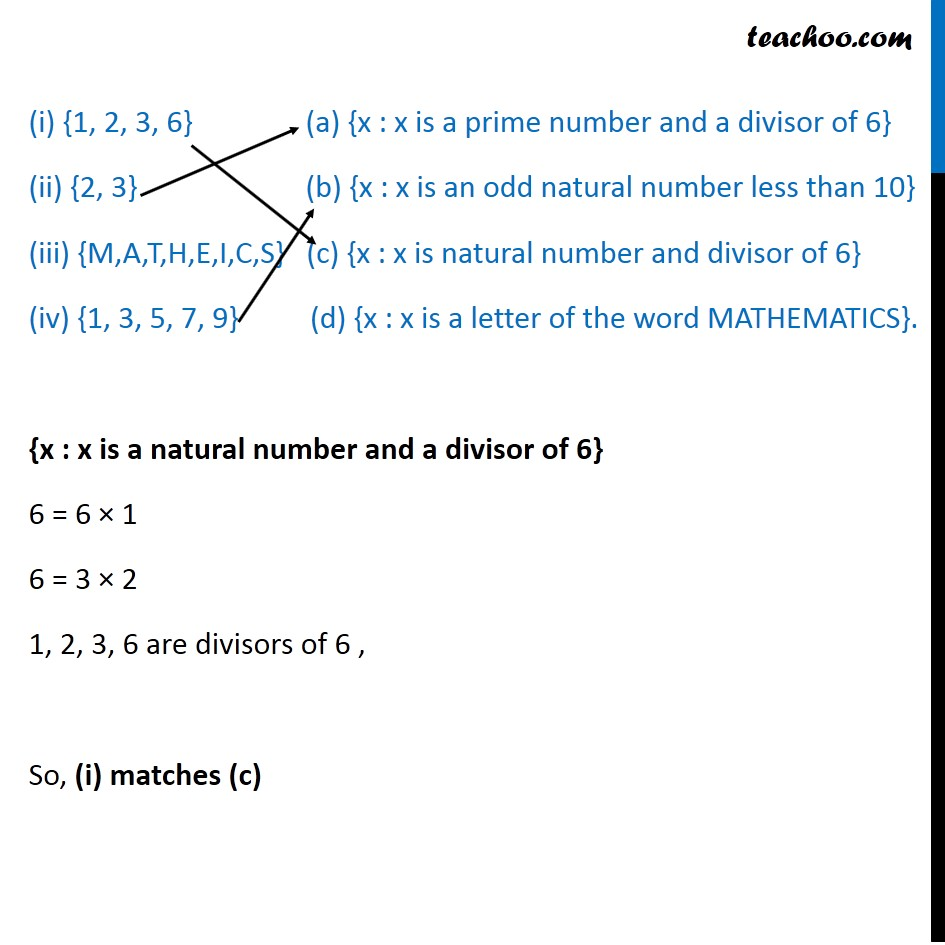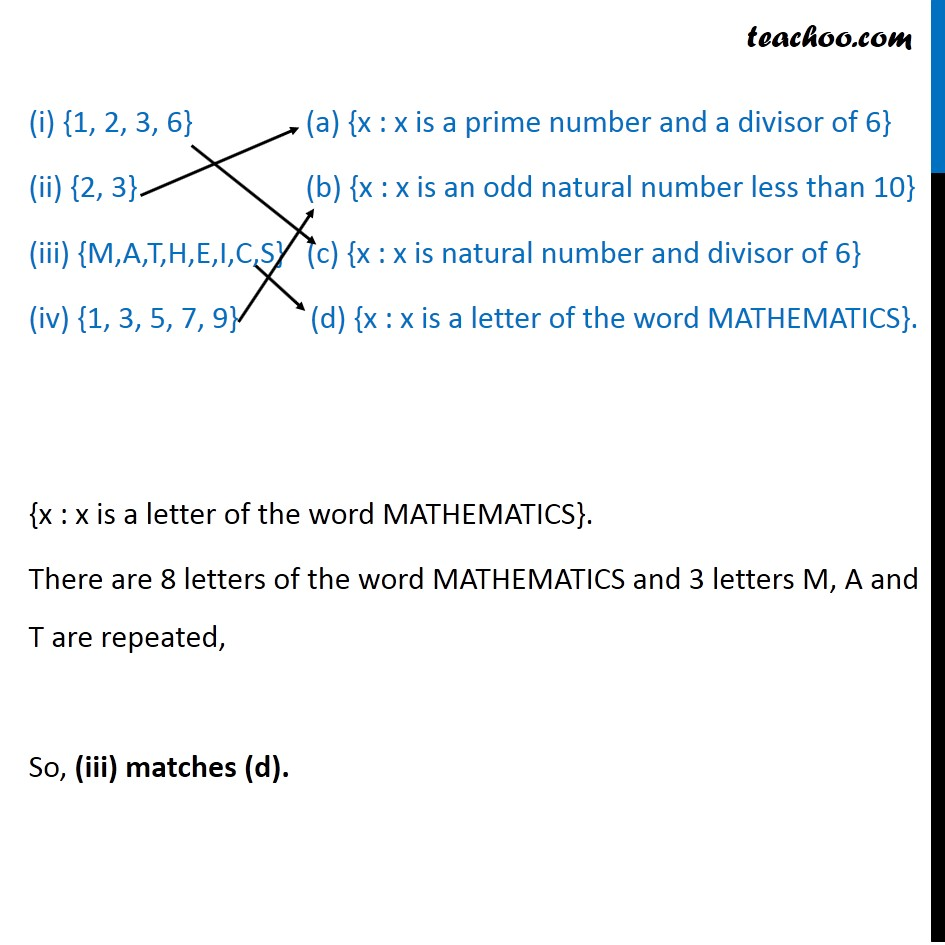1. Chapter 1 Class 11 Sets
2. Concept wise
3. Depicition of sets - Set builder form

Transcript

Ex 1.1, 6 Match each of the set on the left in the roster form with the same set on the right described in set–builder form: (i) {1, 2, 3, 6} (a) {x : x is a prime number and a divisor of 6} (ii) {2, 3} (b) {x : x is an odd natural number less than 10} (iii) {M,A,T,H,E,I,C,S} (c) {x : x is natural number and divisor of 6} (iv) {1, 3, 5, 7, 9} (d) {x : x is a letter of the word MATHEMATICS}. {x : x is a prime number and a divisor of 6} 6 = 6 × 1 6 = 3 × 2 1, 2, 3, 6 are divisors of 6 , out of which 2, 3 are prime So, (ii) matches (a) (i) {1, 2, 3, 6} (a) {x : x is a prime number and a divisor of 6} (ii) {2, 3} (b) {x : x is an odd natural number less than 10} (iii) {M,A,T,H,E,I,C,S} (c) {x : x is natural number and divisor of 6} (iv) {1, 3, 5, 7, 9} (d) {x : x is a letter of the word MATHEMATICS}. {x : x is an odd natural number less than 10} Natural numbers are 1, 2, 3, 4, 5, 6, 7, 8,… Odd Natural numbers are 1, 3, 5, 7, 9, 11, 13 Odd natural numbers less than 10 are 1, 3, 5, 7, 9 Hence, (iv) matches (b) (i) {1, 2, 3, 6} (a) {x : x is a prime number and a divisor of 6} (ii) {2, 3} (b) {x : x is an odd natural number less than 10} (iii) {M,A,T,H,E,I,C,S} (c) {x : x is natural number and divisor of 6} (iv) {1, 3, 5, 7, 9} (d) {x : x is a letter of the word MATHEMATICS}. {x : x is a natural number and a divisor of 6} 6 = 6 × 1 6 = 3 × 2 1, 2, 3, 6 are divisors of 6 , So, (i) matches (c) (i) {1, 2, 3, 6} (a) {x : x is a prime number and a divisor of 6} (ii) {2, 3} (b) {x : x is an odd natural number less than 10} (iii) {M,A,T,H,E,I,C,S} (c) {x : x is natural number and divisor of 6} (iv) {1, 3, 5, 7, 9} (d) {x : x is a letter of the word MATHEMATICS}. {x : x is a letter of the word MATHEMATICS}. There are 8 letters of the word MATHEMATICS and 3 letters M, A and T are repeated, So, (iii) matches (d).

Depicition of sets - Set builder form